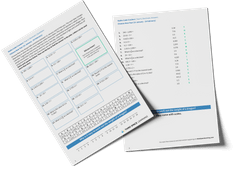Math teaching support you can trustone-on-one tutoring sessionsschools supported

[FREE] Fun Math Games & Activities Packs

Always on the lookout for fun math games and activities in the classroom? Try our ready-to-go printable packs for students to complete independently or with a partner!

# What Are Coordinates: Explained For Teachers, Parents And Kids

Learning what coordinates are and how they work can be one of the most confusing parts of elementary and middle school math. That’s why we’ve put together this guide to help you explain coordinates more easily to your children!

This blog is part of our series of blogs designed for teachers, schools and parents supporting home learning and looking for free home learning resources during the Covid-19 pandemic.

### What are coordinates?

Coordinates are two numbers (Cartesian coordinates), or sometimes a letter and a number, that locate a specific point on a grid, known as a coordinate plane. A coordinate plane has four quadrants and two axes: the x-axis (horizontal) and y-axis (vertical). (One way of helping children to remember which of these is which is to tell them that the x-axis goes across because ‘x’ is a cross.)

Children are introduced to coordinates in the first quadrant (the top right quadrant) as both coordinate digits will be positive. The point at which the two axes intersect is called the origin – the coordinates of this point are (0, 0).

Coordinates are written as (x, y) meaning the point on the x-axis is written first, followed by the point on the y-axis.

Some children may be taught to remember this with the phrase ‘along the hall, up the stairs’, meaning that they should follow the x-axis first and then the y.

### When will my child learn about coordinates in elementary school?

Schools following Common Core:

Coordinates are first taught in 5th Grade, where children learn to use graph points on the coordinate plane to solve real-world and mathematical problems and to classify two-dimensional figures into categories based on their properties.

• 5th Grade: Students can identify the parts of a coordinate plane and plot a given point on the plane using ordered pairs in the first quadrant. Students can also represent and interpret real-world and math problems by graphing points on the coordinate plane.
• 6th Grade: Students extend coordinate axes from previous grades to represent points on the line and in the plane with negative number coordinates. Students solve real-world and math problems by graphing points in all four quadrants of the coordinate plane. Students also find distances between points with the same first coordinate or the same second coordinate. Finally, students draw polygons in the coordinate plane given coordinates for the vertices and using coordinates to find the length of a side with the same first coordinate or the same second coordinate.

The teaching of coordinates ends in 6th grade, where children learn to describe positions on the full coordinate grid (all four quadrants) and draw and translate simple shapes on the coordinate plane and reflect them in the axes.

Other schools:

Schools not following Common Core also teach the coordinate plane in 5th and 6th grades, although specific standards may differ slightly.

### How do coordinates relate to other areas of math?

Coordinates sometimes appear in other areas of math, specifically graphs and algebra. Older students connect their work on coordinates and scales to their interpretation of time graphs and they should be introduced to the use of symbols and letters to represent variables and unknowns in mathematical situations that they already understand, such as coordinates.

Wondering about how to explain other key math vocabulary to your children? Check out our Math Dictionary For Kids, or try these other terms related to coordinates:

### Practice questions

1. Layla draws a square on this coordinate grid. Three of the vertices are marked. What are the coordinates of the missing vertex?

2. In this diagram, R is an equal distance from P and Q. What are the coordinates of R?

3. This diagram shows two identical rectangles on coordinate axes. Write the coordinates of point A and point B.

(Answer: A = 12, 6 & B = 19, 3)

4. Here is a kite. Write the coordinates of point D.

5. A counter is placed on square D4. It is moved 2 squares right and 3 squares down. Write the position of the square it lands on.

Do you have students who need extra support in math?
Give your students more opportunities to consolidate learning and practice skills through personalized math tutoring with their own dedicated online math tutor.

Each student receives differentiated instruction designed to close their individual learning gaps, and scaffolded learning ensures every student learns at the right pace. Lessons are aligned with your state’s standards and assessments, plus you’ll receive regular reports every step of the way.

Personalized one-on-one math tutoring programs are available for:

The content in this article was originally written by primary school teacher Sophie Bartlett and has since been revised and adapted for US schools by elementary math teacher Katie Keeton.##### Sophie Bartlett
Year 6 Teacher
Sophie teaches mixed age classes at a small school in central England. She is a self confessed grammar pedant and number nerd so we've welcomed her as a regular author and editor for Third Space Learning.
xx

#### [FREE] Fun Math Games & Activities Packs

Individual packs containing fun math games and activities to complete in the classroom.

The activities are designed to be fun, flexible and suitable for a range of abilities.